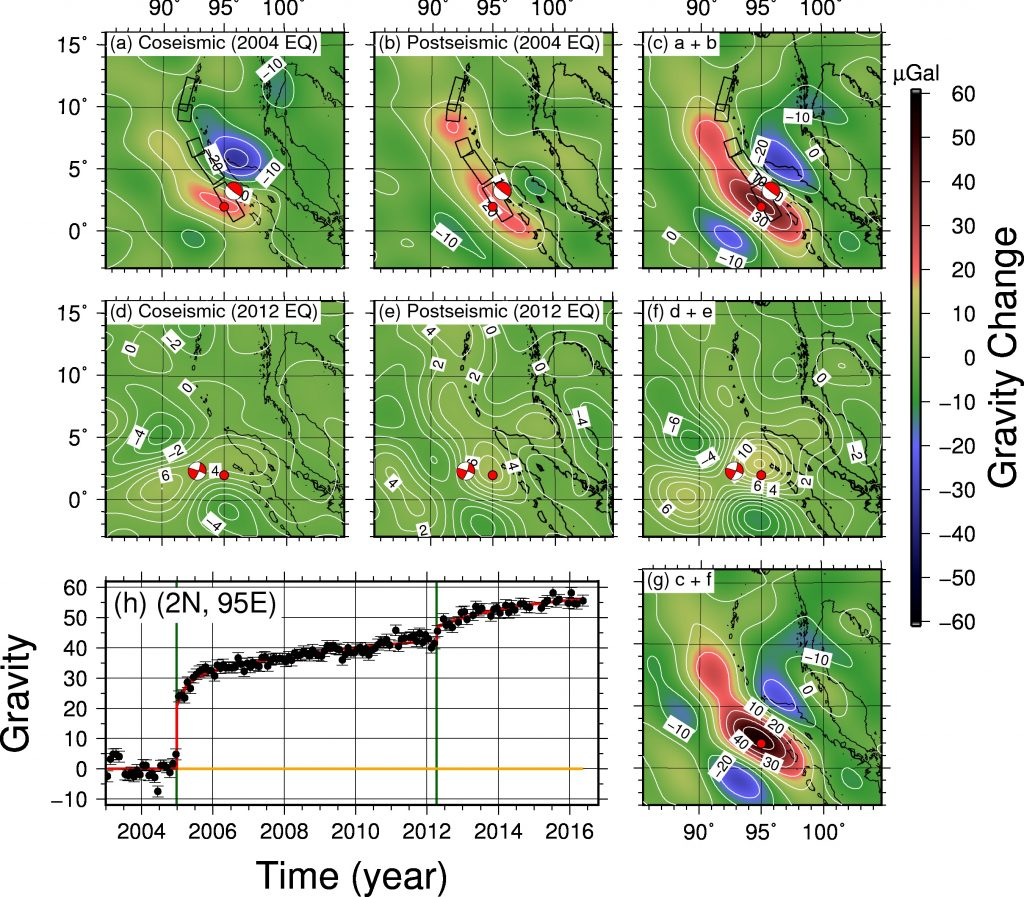#### Gravity and Geoid Changes by the 2004 and 2012 Sumatra Earthquakes from Satellite Gravimetry and Ocean AltimetryYusaku Tanaka1, Yao Yu2, 3, and Benjamin Fong Chao3
1. Earthquake Research Institute, The University of Tokyo, Tokyo, Japan
2. School of Geodesy and Geomatics, Wuhan University, Wuhan, China
3. Institute of Earth Science, Academia Sinica, Taipei, Taiwan

Terrestrial, Atmospheric and Oceanic Sciences, Vol. 30, No. 2, 1-10

Abstract
We first report the results from analyzing the GRACE satellite data for the coseismic and long-term post-seismic changes in the Earth’s gravity field and geoid height induced by the 2004 Sumatra-Andaman earthquake (Mw 9.2) and the 2012 Indian-Ocean earthquake (Mw 8.6). The results suggest that the two earthquakes have changed the gravity field and geoid height irreversibly (as opposed to cyclically). We next use satellite ocean altimetry measurements to search for the geoid height change induced by the Sumatra-Andaman earthquake, employing an effective method of extracting coherent space-temporal signals, namely the empirical orthogonal functions (EOF) analysis. Our results demonstrate that the sea level variation in the studied (tropical) area during the studied (10-year) period is dominated by the strong steric changes related to ENSO, to the point that the earthquake-induced signals, even using EOF for the largest earthquakes, are obscured and thus undetectable by ocean altimetry. However, this also means that steric and non-steric changes can be observed separately from artificial satellites.Fig. 2. (a) Coseismic gravity change (in μGal) induced by the 2004 Sumatra-Andaman earthquake (its rectangular faults are depicted). (b) The sum of short- and long-term post-seismic gravity changes from the earthquake occurrences till May 2016. (c) The sum of (a) + (b), i.e., co- and postseismic gravity changes. (d), (e), (f) The same as (a), (b), (c) but for the 2012 Sumatra/Indian-Ocean earthquake. (g) The total gravity changes by the two earthquakes. The fault mechanisms of the two earthquakes are shown at the epicenters. (h) The time series of gravity changes Δg at the red circles marked in (a) – (g). The overall mean and average seasonal changes have been removed. The black dots with error bars (assumed uniform in time and have been scaled with post-fit residuals) show monthly gravity data and the red curve is the least-squares fit. The two vertical green lines indicates the occurrences of the two earthquakes.